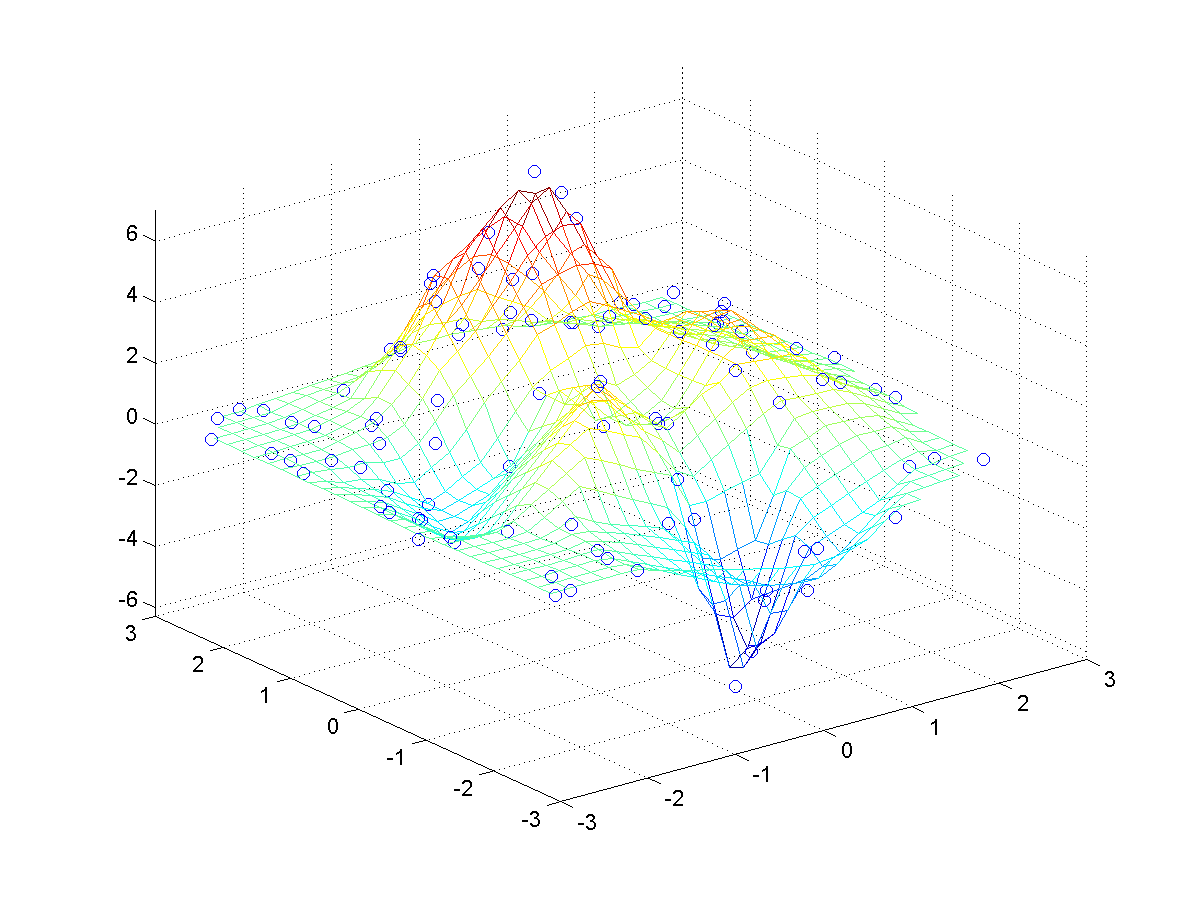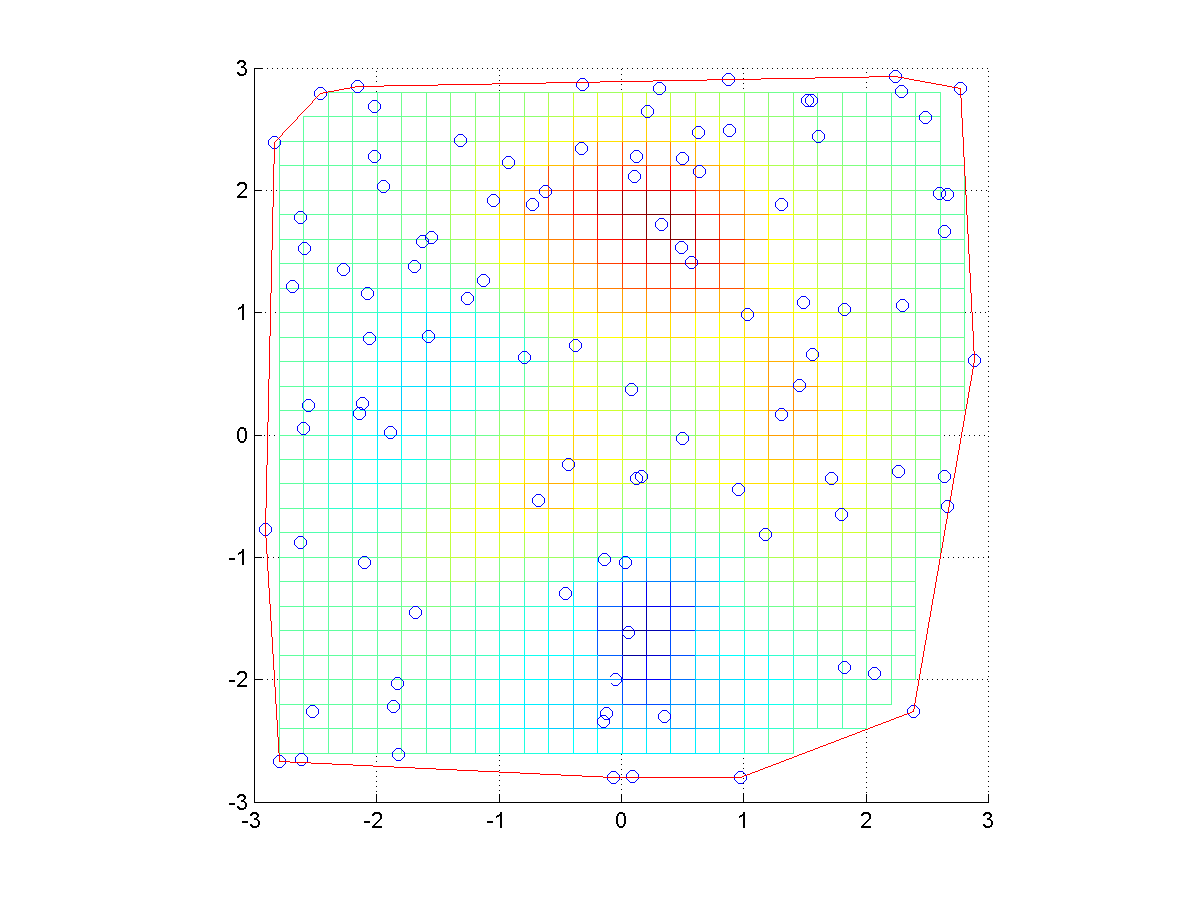## 9-3 嚙踝蕭嚙踝蕭嚙踝蕭嚙踝蕭嚙踝蕭嚙踝蕭嚙踝蕭嚙踝蕭嚙踝蕭嚙踝蕭嚙踝蕭嚙踝蕭

zi = griddata(x, y, z, xi, yi)

Example 1: 09-內插法/griddata01.mx = 6*rand(100, 1)-3; % [-3, 3] 之間的 100 個均勻分佈亂數 y = 6*rand(100, 1)-3; % [-3, 3] 之間的 100 個均勻分佈亂數 z = peaks(x, y); [xi, yi] = meshgrid(-3:0.2:3, -3:0.2:3); zi = griddata(x, y, z, xi, yi); mesh(xi, yi, zi); % 畫出曲面 hold on; plot3(x, y, z, 'o'); hold off % 畫出資料點 axis tight; hidden off; figure; mesh(xi, yi, zi); view(2); axis image % 曲面俯視圖 hold on; plot3(x, y, z+1, 'o'); hold off % 畫出資料點 k = convhull(x, y); line(x(k), y(k), 10*ones(1,length(k)), 'color', 'r'); % Add convex hull• 在第一個圖中，每一圓球代表取樣點（共 100 點），而曲面則是使用 griddata 指令的內插結果。
• griddata 指令只進行內插，而不進行外插，因此所產生的曲面資料只定義在這些取樣點所形成的最小凸多邊型（Convex Hull），如第二個圖所示。

MATLAB程式設計：進階篇